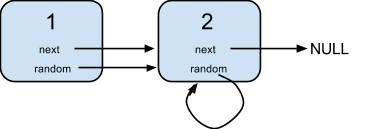### LeetCode• ㊗️
• 大家
• offer
• 多多！

## Problem

A linked list is given such that each node contains an additional random pointer which could point to any node in the list or null.

Return a deep copy of the list.

Example 1:Input:
{"$id":"1","next":{"$id":"2","next":null,"random":{"$ref":"2"},"val":2},"random":{"$ref":"2"},"val":1}

Explanation:
Node 1's value is 1, both of its next and random pointer points to Node 2.
Node 2's value is 2, its next pointer points to null and its random pointer points to itself.


Note:

You must return the copy of the given head as a reference to the cloned list.

## Code

/*
// Definition for a Node.
class Node {
public int val;
public Node next;
public Node random;

public Node() {}

public Node(int _val,Node _next,Node _random) {
val = _val;
next = _next;
random = _random;
}
};
*/
class Solution {
HashMap<Node, Node> map = new HashMap<>();

while(curr != null){
map.put(curr, new Node(curr.val));
curr = curr.next;
}